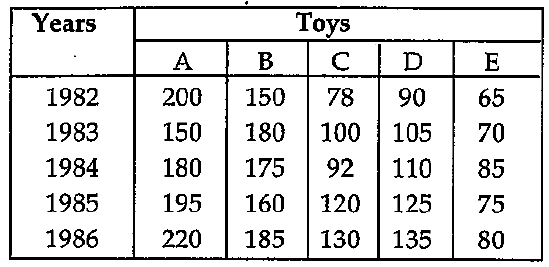# Numerical Reasoning - Numerical Test 1

Instructions:

Study the following table carefully and answer the questionsgievn below it:

1:

What was the percentage (approximate) increase in production of D type of toys from 1983 to 1985?

 A. 10 B. 20 C. 19 D. 25 Answer Report Discuss Option: C Explanation : Production of D type toys in 1983 = 1,05,000 Production of D type toys in 1985 = 1,25,000 Percentage increase = (20000/105000) * 100 = 19.05 = 19% (app.) Click on Discuss to view users comments. Write your comments here:
2:

In the case of which type of toys was the total production of the given five years the maximum?

 A. C B. B C. A D. D Answer Report Discuss Option: C Explanation : Total production of A types toys from 1982-86 = 945 thousands Total production of B types toys from 1982-86 = 850 thousands Total production of C types toys from 1982-86 = 520 thousands Total production of D types toys from 1982-86 = 565 thousands Total production of E types toys from 1982-86 = 375 thousands Hence it was maximum for A type toys. Click on Discuss to view users comments. Write your comments here:
3:

What was the percentage drop on production of A type toys from 1982 to 1984?

 A. 30 B. 10 C. 20 D. 50 Answer Report Discuss Option: B Explanation : Drop in production A type toys from 1982 to 1984 = 20000 Percentage drop = (20000/200000) * 100 = 10%   Click on Discuss to view users comments. Write your comments here:
4:

In the case of which type of toys was there a continuous increase in production over the years?

 A. B B. A C. C D. D Answer Report Discuss Option: D Explanation : From the table it is obvious that there is a continuous increaes in production of D type of toys Click on Discuss to view users comments. Write your comments here:
5:

The production of E type of toys in 1986 was what percent of production of B type toys in 1985?

 A. 80 B. 50 C. 100 D. 200 Answer Report Discuss Option: B Explanation : If the required number is x, then by hypothesis 80,000 = x% of 1,60,000 or 80,000 = (x/100) * 160000 Therefore x = (80000 *100)/160000 = 50 Click on Discuss to view users comments. Write your comments here:

## Suggest an improvement

1. Take a free Numerical test before your next interview.
2. Take a numeracy psychometric test designed especially for CES and USB
3. Here you can practice questions for ceb numerical reasoning test.
4. These questions can be used for investment banking numerical test .
5. Here you will find questions answers for numeracy psychometric test.
6. This section provides you free online psychometric test.
7. These questions can be used for numerical assessment